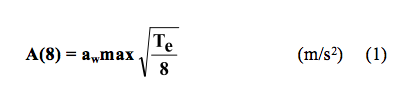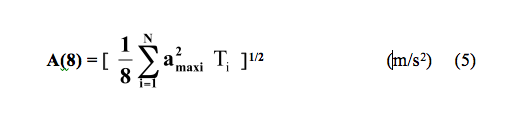POSIZIONE: PAF > Vibrazioni Corpo Intero > EsposizioneRegione Toscana Diritti Valori Innovazione SostenibilitÃServizio Sanitario della Toscana

# Calculating the level of exposure

The current legislation prescribes that the level of exposure to vibration is assessed by the calculation of the frequency-weighted equivalent acceleration value over an eight-hour reference period. This can be calculated by using the following formula:where:

Te :         Total daily duration of exposure to the vibration (hours)

avmax    Highest of the three orthogonal axes acceleration values:

### awmax(m/s2) = MAX( 1,4 awx ; 1,4 awy ; awz )

If the worker is exposed to different vibration values, as in the case of  the usage of several machines throughout the working day, the daily vibration exposure A(8) expressed as m/s2, will be calculated as follows:where:

a2maxi : frequency-weighted acceleration for the i-th operation

Ti: Exposure duration for the i-th operation (hours)